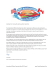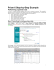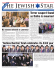# 12-4 Volumes of Prisms and Cylinders

## Transcription

12-4 Volumes of Prisms and Cylinders
```12-4 Volumes of Prisms and Cylinders
Find the volume of each prism.
62/87,21
The volume V of a prism is V = Bh, where B is the area of a base and h LVWKHKHLJKWRIWKHSULVP
3
The volume is 108 cm .
62/87,21
The volume V of a prism is V = Bh, where B is the area of a base and h LVWKHKHLJKWRIWKHSULVP
the oblique rectangular prism shown at the right
62/87,21
If two solids have the same height h and the same cross-sectional area B at every level, then they have the same
volume. So, the volume of a right prism and an oblique one of the same height and cross sectional area are same.
Page 1
12-4 Volumes of Prisms and Cylinders
the oblique rectangular prism shown at the right
62/87,21
If two solids have the same height h and the same cross-sectional area B at every level, then they have the same
volume. So, the volume of a right prism and an oblique one of the same height and cross sectional area are same.
an oblique pentagonal prism with a base area of 42 square centimeters and a height of 5.2 centimeters
62/87,21
If two solids have the same height h and the same cross-sectional area B at every level, then they have the same
volume. So, the volume of a right prism and an oblique one of the same height and cross sectional area are same.
Find the volume of each cylinder. Round to the nearest tenth.
62/87,21
62/87,21
If two solids have the same height h and the same cross-sectional area B at every level, then they have the same
volume.
So,
the volume
of a right cylinder and an oblique one of the same height and cross sectional area are same.
eSolutions
Manual
- Powered
by Cognero
Page 2
12-4 Volumes of Prisms and Cylinders
62/87,21
If two solids have the same height h and the same cross-sectional area B at every level, then they have the same
volume. So, the volume of a right cylinder and an oblique one of the same height and cross sectional area are same.
a cylinder with a diameter of 16 centimeters and a height of 5.1 centimeters
62/87,21
a cylinder with a radius of 4.2 inches and a height of 7.4 inches
62/87,21
MULTIPLE CHOICE A rectangular lap pool measures 80 feet long by 20 feet wide. If it needs to be filled to four
feet deep and each cubic foot holds 7.5 gallons, how many gallons will it take to fill the lap pool?
A 4000
B 6400
C 30,000
D 48,000
62/87,21
(DFKFXELFIRRWKROGVJDOORQVRIZDWHU6RWKHDPRXQWRIZDWHUUHTXLUHGWRILOOWKHSRROLV Therefore, the correct choice is D.
Find the volume of each prism.
Page 3
(DFKFXELFIRRWKROGVJDOORQVRIZDWHU6RWKHDPRXQWRIZDWHUUHTXLUHGWRILOOWKHSRROLV 12-4 Volumes of Prisms and Cylinders
Therefore, the correct choice is D.
Find the volume of each prism.
62/87,21
7KHEDVHLVDUHFWDQJOHRIOHQJWKLQDQGZLGWKLQ7KHKHLJKWRIWKHSULVPLVLQ
62/87,21
The base is a triangle with a base length of 11 m and the corresponding height of 7 m. The height of the prism is 14
P
62/87,21
The base is a right triangle with a leg length of 9 cm and the hypotenuse of length 15 cm.
Use the Pythagorean Theorem to find the height of the base.
7KHKHLJKWRIWKHSULVPLVFP
Page 4
12-4 Volumes of Prisms and Cylinders
62/87,21
The base is a right triangle with a leg length of 9 cm and the hypotenuse of length 15 cm.
Use the Pythagorean Theorem to find the height of the base.
7KHKHLJKWRIWKHSULVPLVFP
62/87,21
If two solids have the same height h and the same cross-sectional area B at every level, then they have the same
volume. So, the volume of a right prism and an oblique one of the same height and cross sectional area are same.
an oblique hexagonal prism with a height of 15 centimeters and with a base area of 136 square centimeters
62/87,21
If two solids have the same height h and the same cross-sectional area B at every level, then they have the same
volume. So, the volume of a right prism and an oblique one of the same height and cross sectional area are same.
Page 5
12-4Volumes of Prisms and Cylinders
an oblique hexagonal prism with a height of 15 centimeters and with a base area of 136 square centimeters
62/87,21
If two solids have the same height h and the same cross-sectional area B at every level, then they have the same
volume. So, the volume of a right prism and an oblique one of the same height and cross sectional area are same.
a square prism with a base edge of 9.5 inches and a height of 17 inches
62/87,21
If two solids have the same height h and the same cross-sectional area B at every level, then they have the same
volume. So, the volume of a right prism and an oblique one of the same height and cross sectional area are same.
Find the volume of each cylinder. Round to the nearest tenth.
62/87,21
r = 5 yd and h = 18 yd
62/87,21
r = 6 cm and h FP
Page 6
12-4 Volumes of Prisms and Cylinders
62/87,21
r = 6 cm and h FP
62/87,21
r LQ
Use the Pythagorean Theorem to find the height of the cylinder.
Now you can find the volume.
62/87,21
If two solids have the same height h and the same cross-sectional area B at every level, then they have the same
volume.
So,
the volume
of a right prism and an oblique one of the same height and cross sectional area are same.Page 7
eSolutions
Manual
- Powered
by Cognero
r = 7.5 mm and h PP
12-4 Volumes of Prisms and Cylinders
62/87,21
If two solids have the same height h and the same cross-sectional area B at every level, then they have the same
volume. So, the volume of a right prism and an oblique one of the same height and cross sectional area are same.
r = 7.5 mm and h PP
PLANTER A planter is in the shape of a rectangular prism 18 inches long,
What is the volume of potting soil in the planter if the planter is filled to
inches deep, and 12 inches high.
inches below the top?
62/87,21
The planter is to be filled
inches below the top, so
SHIPPING A box 18 centimeters by 9 centimeters by 15 centimeters is being used to ship two cylindrical candles.
Each candle has a diameter of 9 centimeters and a height of 15 centimeters, as shown at the right. What is the
volume of the empty space in the box?
62/87,21
The volume of the empty space is the difference of volumes of the rectangular prism and the cylinders.
eSolutions
Page 8
SANDCASTLES In a sandcastle competition, contestants are allowed to use only water, shovels, and 10 cubic feet
12-4 Volumes of Prisms and Cylinders
SHIPPING A box 18 centimeters by 9 centimeters by 15 centimeters is being used to ship two cylindrical candles.
Each candle has a diameter of 9 centimeters and a height of 15 centimeters, as shown at the right. What is the
volume of the empty space in the box?
62/87,21
The volume of the empty space is the difference of volumes of the rectangular prism and the cylinders.
SANDCASTLES In a sandcastle competition, contestants are allowed to use only water, shovels, and 10 cubic feet
of sand. To transport the correct amount of sand, they want to create cylinders that are 2 feet tall to hold enough
sand for one contestant. What should the diameter of the cylinders be?
62/87,21
V = 10 ft3 and h = 2 ft Use the formula to find r.
Therefore, the diameter of the cylinders should be about 2.52 ft.
Find the volume of the solid formed by each net.
62/87,21
The middle piece of the net is the front of the solid. The top and bottom pieces are the bases and the pieces on the
ends are the side faces. This is a triangular prism.
One leg of the base 14 cm and the hypotenuse 31.4 cm. Use the Pythagorean Theorem to find the height of the
base.
Page 9
12-4Volumes of Prisms and Cylinders
Therefore, the diameter of the cylinders should be about 2.52 ft.
Find the volume of the solid formed by each net.
62/87,21
The middle piece of the net is the front of the solid. The top and bottom pieces are the bases and the pieces on the
ends are the side faces. This is a triangular prism.
One leg of the base 14 cm and the hypotenuse 31.4 cm. Use the Pythagorean Theorem to find the height of the
base.
7KHKHLJKWRIWKHSULVPLVFP
The volume V of a prism is V = Bh, where B is the area of the base, h LVWKHKHLJKWRIWKHSULVP
62/87,21
The circular bases at the top and bottom of the net indicate that this is a cylinder. If the middle piece were a
rectangle, then the prism would be right. However, since the middle piece is a parallelogram, it is oblique.
The radius is 1.8 m, the height is 4.8 m, and the slant height is 6 m.
If two solids have the same height h and the same cross-sectional area B at every level, then they have the same
volume. So, the volume of a right prism and an oblique one of the same height and cross sectional area are same.
Page 10
FOOD A cylindrical can of baked potato chips has a height of 27 centimeters and a radius of 4 centimeters. A new
12-4 Volumes of Prisms and Cylinders
62/87,21
The circular bases at the top and bottom of the net indicate that this is a cylinder. If the middle piece were a
rectangle, then the prism would be right. However, since the middle piece is a parallelogram, it is oblique.
The radius is 1.8 m, the height is 4.8 m, and the slant height is 6 m.
If two solids have the same height h and the same cross-sectional area B at every level, then they have the same
volume. So, the volume of a right prism and an oblique one of the same height and cross sectional area are same.
FOOD A cylindrical can of baked potato chips has a height of 27 centimeters and a radius of 4 centimeters. A new
can is advertised as being 30% larger than the regular can. If both cans have the same radius, what is the height of
the larger can?
62/87,21
The volume of the smaller can is
7KHYROXPHRIWKHQHZFDQLVRIWKHVPDOOHUFDQZLWKWKHVDPHUDGLXV
The height of the new can will be 35.1 cm.
CHANGING DIMENSIONS A cylinder has a radius of 5 centimeters and a height of 8 centimeters. Describe
Page 11
of the cylinder.
a. The height is tripled.
eSolutions
- Powered
by Cognero
howManual
each change
affects
the volume
height of
of Prisms
the new and
can will
be 35.1 cm.
12-4The
Volumes
Cylinders
CHANGING DIMENSIONS A cylinder has a radius of 5 centimeters and a height of 8 centimeters. Describe
how each change affects the volume of the cylinder.
a. The height is tripled.
c. Both the radius and the height are tripled.
d. The dimensions are exchanged.
62/87,21
a.
When the height is tripled, h = 3h .
When the height is tripled, the volume is multiplied by 3.
b. When the radius is tripled, r = 3r.
So, when the radius is tripled, the volume is multiplied by 9.
c. When the height and the radius are tripled, r = 3r and h = 3h .
:KHQWKHKHLJKWDQGWKHUDGLXVDUHWULSOHGWKHYROXPHLVPXOWLSOLHGE\
d. When the dimensions are exchanged, r = 8 and h = 5 cm.
Compare to the original volume.
Page 12
12-4:KHQWKHKHLJKWDQGWKHUDGLXVDUHWULSOHGWKHYROXPHLVPXOWLSOLHGE\
Volumes of Prisms and Cylinders
d. When the dimensions are exchanged, r = 8 and h = 5 cm.
Compare to the original volume.
The volume is multiplied by
.
The prisms described below have the same height as the prism shown. Which of the three prisms has the same
volume as this prism? Explain your reasoning.
Prism A: The base is a right triangle with legs 8 inches and 5 inches.
Prism B: The base is a square with side lengths of 4.5 inches.
Prism C: The base is a hexagon with side lengths of 3 inches.
62/87,21
The volume of a prism is given by
. Prisms that have the same height h will have equal volumes if their
bases have equal areas. All the prisms have a height of h inches. Find the area of the base of Prism A, B, and C and
compare to the given prism.
Prism A:
7KHEDVHLVDULJKWWULDQJOHZLWKOHJVLQFKHVDQGLQFKHV)LQGWKHDUHDRIWKLVEDVH
So, the area of the base of Prism A is 20 in2.
Prism B:
7KHEDVHLVDVTXDUHZLWKVLGHVRILQFKHV)LQGWKHDUHDRIWKLVEDVH
Page 13
12-4 Volumes of Prisms and Cylinders
7KHEDVHLVDVTXDUHZLWKVLGHVRILQFKHV)LQGWKHDUHDRIWKLVEDVH
So, the area of the base of Prism B is 20.25 in2.
Prism C:
The base is a regular hexagon with sides of 3 inches. First, find the measure of the apothem and then find the area of
WKLVEDVH
A central angle of the hexagon is
RUVRWKHDQJOHIRUPHGLQWKHWULDQJOHEHORZLV
Use a trigonometric ratio to find the apothem a and then find the area of this base.
So, the area of the base of Prism C is about 23.4 in2.
7KHEDVHRIWKHSULVPVKRZQLQWKHSUREOHPLVDUHFWDQJOHZLWKDQDUHDHTXDOWRîRULQwhich is the same
as Prism A.
Therefore, Prism A has the same volume as the given rectangular prism.
Find the volume of each composite solid. Round to the nearest tenth if necessary.
Page 14
So, the area of the base of Prism C is about 23.4 in2.
7KHEDVHRIWKHSULVPVKRZQLQWKHSUREOHPLVDUHFWDQJOHZLWKDQDUHDHTXDOWRîRULQwhich is the same
12-4 Volumes of Prisms and Cylinders
as Prism A.
Therefore, Prism A has the same volume as the given rectangular prism.
Find the volume of each composite solid. Round to the nearest tenth if necessary.
62/87,21
The solid is a combination of two rectangular prisms. The base of one rectangular prism is 5 cm by 3 cm and the
KHLJKWLVFP7KHEDVHRIWKHRWKHUSULVPLVFPE\FPDQGWKHKHLJKWLVFP
62/87,21
The solid is a combination of a rectangular prism and a right triangular prism. The total volume of the solid is the sum
of the volumes of the two rectangular prisms.
62/87,21
7KHVROLGLVDFRPELQDWLRQRIDUHFWDQJXODUSULVPDQGWZRKDOIF\OLQGHUV
Page 15
12-4 Volumes of Prisms and Cylinders
62/87,21
7KHVROLGLVDFRPELQDWLRQRIDUHFWDQJXODUSULVPDQGWZRKDOIF\OLQGHUV
MANUFACTURING A can 12 centimeters tall fits into a rubberized cylindrical holder that is 11.5 centimeters tall,
including 1 centimeter for the thickness of the base of the holder. The thickness of the rim of the holder is 1
centimeter. What is the volume of the rubberized material that makes up the holder?
62/87,21
The volume of the rubberized material is the difference between the volumes of the container and the space used for
the can. The container has a radius of
DQGDKHLJKWRIFP7KHHPSW\VSDFHXVHGWRNHHSWKH
2
can has a radius of 3.25 cm and a height of 11.5 ± 1 = 10.5 cm. The volume V of a cylinder is V = ʌr h ZKHUHr is
the radius of the base and h LVWKHKHLJKWRIWKHF\OLQGHU
3
Therefore, the volume of the rubberized material is about 304.1 cm .
Find each measure to the nearest tenth.
A cylindrical can has a volume of 363 cubic centimeters. The diameter of the can is 9 centimeters. What is the
height?
62/87,21
Page 16
12-4 Volumes of Prisms and Cylinders
3
Therefore, the volume of the rubberized material is about 304.1 cm .
Find each measure to the nearest tenth.
A cylindrical can has a volume of 363 cubic centimeters. The diameter of the can is 9 centimeters. What is the
height?
62/87,21
A cylinder has a surface area of 144ʌsquare inches and a height of 6 inches. What is the volume?
62/87,21
Use the surface area formula to solve for r.
The radius is 6. Find the volume.
A rectangular prism has a surface area of 432 square inches, a height of 6 inches, and a width of 12 inches. What is
the volume?
62/87,21
Use the surface area formula to find the length of the base of the prism.
eSolutions
Page 17
12-4Volumes of Prisms and Cylinders
A rectangular prism has a surface area of 432 square inches, a height of 6 inches, and a width of 12 inches. What is
the volume?
62/87,21
Use the surface area formula to find the length of the base of the prism.
Find the volume.
ARCHITECTURE A cylindrical stainless steel column is used to hide a ventilation system in a new building.
According to the specifications, the diameter of the column can be between 30 centimeters and 95 centimeters. The
height is to be 500 centimeters. What is the difference in volume between the largest and smallest possible column?
Round to the nearest tenth cubic centimeter.
62/87,21
The volume will be the highest when the diameter is 95 cm and will be the lowest when it is 30 cm.That is when the
UDGLLDUHFPDQGFPUHVSHFWLYHO\
Find the difference between the volumes.
SWIMMING POOLS The base of a rectangular swimming pool is sloped so one end of the pool is 6 feet deep and
the other end is 3 feet deep, as shown in the figure. If the width is 15 feet, find the volume of water it takes to fill the
pool.
62/87,21
eSolutions
- Powered
The Manual
swimming
poolbyisCognero
a combination
of a rectangular prism and a trapezoidal prism. The base of the rectangularPage 18
prism is 6 ft by 10 ft and the height is 15 ft. The bases of the trapezoidal prism are 6 ft and 3 ft long and the height of
the base is 10 ft. The height of the trapezoidal prism is 15 ft. The total volume of the solid is the sum of the volumes
12-4 Volumes of Prisms and Cylinders
SWIMMING POOLS The base of a rectangular swimming pool is sloped so one end of the pool is 6 feet deep and
the other end is 3 feet deep, as shown in the figure. If the width is 15 feet, find the volume of water it takes to fill the
pool.
62/87,21
The swimming pool is a combination of a rectangular prism and a trapezoidal prism. The base of the rectangular
prism is 6 ft by 10 ft and the height is 15 ft. The bases of the trapezoidal prism are 6 ft and 3 ft long and the height of
the base is 10 ft. The height of the trapezoidal prism is 15 ft. The total volume of the solid is the sum of the volumes
of the two prisms.
CHANGING DIMENSIONS A soy milk company is planning a promotion in which the volume of soy milk in each
container will be increased by 25%. The company wants the base of the container to stay the same. What will be the
height of the new containers?
62/87,21
Find the volume of the original container.
The volume of the new container is 125% of the original container, with the same base dimensions. Use 1.25V and B
to find h .
eSolutions
Cognero
MEASUREMENT
a real
find the volume.
62/87,21
Page 19
prism or cylinder. Measure its dimensions to the nearest tenth of a centimeter and
12-4 Volumes of Prisms and Cylinders
MEASUREMENT Find a real prism or cylinder. Measure its dimensions to the nearest tenth of a centimeter and
find the volume.
62/87,21
6DPSOHDQVZHU\$FDQRIVRXSLVDF\OLQGHUZLWKDGLDPHWHURIFPDQGDKHLJKWRIFP
3
The volume is about 342.1 cm .
Find the volume of the regular pentagonal prism by dividing it into five equal triangular prisms. Describe the base
area and height of each triangular prism.
62/87,21
The base of the prism can be divided into 5 congruent triangles of a base 8 cm and the corresponding height 5.5 cm.
So, the pentagonal prism is a combination of 5 triangular prisms of height 10 cm. Find the base area of each
triangular prism.
Therefore, the volume of the pentagonal prism is
PATIOS Mr. Thomas is planning to remove an old patio and install a new rectangular concrete patio 20 feet long, 12
feet wide, and 4 inches thick. One contractor bid \$2225 for the project. A second contractor bid \$500 per cubic yard
for the new patio and \$700 for removal of the old patio. Which is the less expensive option? Explain.
62/87,21
Convert all of the dimensions to yards.
20 feet =
yd
12 feet = 4 yd
4 in. =
yd
Find the volume.
eSolutions
Page 20
The total cost for the second contractor is about
.
triangular prism.
12-4 Volumes of Prisms and Cylinders
Therefore, the volume of the pentagonal prism is
PATIOS Mr. Thomas is planning to remove an old patio and install a new rectangular concrete patio 20 feet long, 12
feet wide, and 4 inches thick. One contractor bid \$2225 for the project. A second contractor bid \$500 per cubic yard
for the new patio and \$700 for removal of the old patio. Which is the less expensive option? Explain.
62/87,21
Convert all of the dimensions to yards.
20 feet =
yd
12 feet = 4 yd
4 in. =
yd
Find the volume.
The total cost for the second contractor is about
.
Therefore, the second contractor is a less expensive option.
MULTIPLE REPRESENTATIONS In this problem, you will investigate right and oblique cylinders
.
a. GEOMETRIC Draw a right cylinder and an oblique cylinder with a height of 10 meters and a diameter of 6
meters.
b. VERBAL A square prism has a height of 10 meters and a base edge of 6 meters. Is its volume greater than,
less than, or equal to the volume of the cylinder? Explain.
c. ANALYTICAL Describe which change affects the volume of the cylinder more: multiplying the height by x or
multiplying the radius by x. Explain.
62/87,21
a. The oblique cylinder should look like the right cylinder (same height and size), except that it is pushed a little to the
b. Find the volume of each.
Page 21
12-4 Volumes of Prisms and Cylinders
b. Find the volume of each.
The volume of the square prism is greater.
c. Do each scenario.
2
Assuming x > 1, multiplying the radius by x makes the volume x times greater.
2
For example, if x = 0.5, then x = 0.25, which is less than x.
ERROR ANALYSIS Franciso and Valerie each calculated the volume of an equilateral triangular prism with an
apothem of 4 units and height of 5 units. Is either of them correct? Explain your reasoning.
62/87,21
Francisco; Valerie incorrectly used
DVWKHOHQJWKRIRQHVLGHRIWKHWULDQJXODUEDVH)UDQFLVFRXVHGDGLIIHUHQW
approach, but his solution is correct.
Francisco used the standard formula for the volume of a solid, V = Bh. The area of the base, B, is one-half the
DSRWKHPPXOWLSOLHGE\WKHSHULPHWHURIWKHEDVH
A cylindrical
CHALLENGE
eSolutions
Manual - Powered
by Cognero
can is used to fill a container with liquid. It takes three full cans to fill the container.
Page 22
Describe possible dimensions of the container if it is each of the following shapes.
DSRWKHPPXOWLSOLHGE\WKHSHULPHWHURIWKHEDVH
12-4 Volumes of Prisms and Cylinders
CHALLENGE A cylindrical can is used to fill a container with liquid. It takes three full cans to fill the container.
Describe possible dimensions of the container if it is each of the following shapes.
a. rectangular prism
b. square prism
c. triangular prism with a right triangle as the base
62/87,21
3
The volume of the can is 20ʌin . It takes three full cans to fill the container, so the volume of the container is 60ʌ
3
in .
a. Choose some basic values for 2 of the sides, and then determine the third side. Base: 3 by 5.
3 by 5 by 4ʌ
b. Choose some basic values for 2 of the sides, and then determine the third side. Base: 5 by 5.
5 by 5 by
c. Choose some basic values for 2 of the sides, and then determine the third side. Base: Legs: 3 by 4.
3 by 4 by 10ʌ
Page 23
by 5 by of Prisms and Cylinders
12-45Volumes
c. Choose some basic values for 2 of the sides, and then determine the third side. Base: Legs: 3 by 4.
3 by 4 by 10ʌ
WRITING IN MATH Write a helpful response to the following question posted on an Internet gardening forum.
I am new to gardening. The nursery will deliver a truckload of soil, which they say is 4 yards. I know that a
yard is 3 feet, but what is a yard of soil? How do I know what to order?
62/87,21
3
Sample answer: The nursery means a cubic yard, which is 3 or 27 cubic feet. Find the volume of your garden in
cubic feet and divide by 27 to determine the number of cubic yards of soil needed.
OPEN ENDED Draw and label a prism that has a volume of 50 cubic centimeters.
62/87,21
Choose 3 values that multiply to make 50. The factors of 50 are 2, 5, 5, so these are the simplest values to choose.
REASONING Determine whether the following statement is true or false . Explain.
Two cylinders with the same height and the same lateral area must have the same volume.
62/87,21
True; if two cylinders have the same height (h 1 = h 2) and the same lateral area (L1 = L2), the circular bases must
KDYHWKHVDPHDUHD
The radii must also be equal.
WRITING IN MATH How are the formulas for the volume of a prism and the volume of a cylinder similar? How
are they different?
62/87,21
Both formulas involve multiplying the area of the base by the height. The base of a prism is a polygon, so the
Page 24
expression representing the area varies, depending on the type of polygon it is. The base of a cylinder is a circle, so
2
its area is ʌr .
12-4 Volumes of Prisms and Cylinders
The radii must also be equal.
WRITING IN MATH How are the formulas for the volume of a prism and the volume of a cylinder similar? How
are they different?
62/87,21
Both formulas involve multiplying the area of the base by the height. The base of a prism is a polygon, so the
expression representing the area varies, depending on the type of polygon it is. The base of a cylinder is a circle, so
2
its area is ʌr .
The volume of a triangular prism is 1380 cubic centimeters. Its base is a right triangle with legs measuring 8
centimeters and 15 centimeters. What is the height of the prism?
A 34.5 cm
B 23 cm
C 17 cm
D 11.5 cm
62/87,21
A cylindrical tank used for oil storage has a height that is half the length of its radius. If the volume of the tank is
3
1,122,360 ft , what is the tank¶s radius?
F 89.4 ft
G 178.8 ft
H 280.9 ft
J 561.8 ft
62/87,21
SHORT RESPONSE What is the ratio of the area of the circle to the area of the square?
Page 25
12-4 Volumes of Prisms and Cylinders
SHORT RESPONSE What is the ratio of the area of the circle to the area of the square?
62/87,21
The radius of the circle is 2x and the length of each side of the square is 4x. So, the ratio of the areas can be written
as shown.
SAT/ACT A county proposes to enact a new 0.5% property tax. What would be the additional tax amount for a
landowner whose property has a taxable value of \$85,000?
A \$4.25
B \$170
C \$425
D \$4250
E \$42,500
62/87,21
Find the 0.5% of \$85,000.
Therefore, the correct choice is C.
Find the lateral area and surface area of each regular pyramid. Round to the nearest tenth if necessary.
62/87,21
The lateral area L of a regular pyramid is
, where
LVWKHVODQWKHLJKWDQGP is the perimeter of the base.
The slant height is the height of each of the congruent lateral triangular faces. Use the Pythagorean Theorem to find
the slant height.
eSolutions
Page 26
The lateral area L of a regular pyramid is
, where
LVWKHVODQWKHLJKWDQGP is the perimeter of the base.
12-4Volumes of Prisms and Cylinders
The slant height is the height of each of the congruent lateral triangular faces. Use the Pythagorean Theorem to find
the slant height.
Find the perimeter and area of the equilateral triangle for the base. Use the Pythagorean Theorem to find the height
h of the triangle.
The perimeter is P îRUIHHW
So, the area of the base B is
ft2.
Find the lateral area L and surface area S of the regular pyramid.
Page 27
12-4Volumes of Prisms and Cylinders
So, the area of the base B is
ft2.
Find the lateral area L and surface area S of the regular pyramid.
2
So, the lateral area of the pyramid is about 212.1 ft .
2
Therefore, the surface area of the pyramid is about 255.4 ft .
62/87,21
The lateral area L of a regular pyramid is
, where
LVWKHVODQWKHLJKWDQGP is the perimeter of the base.
Here, the base is a square of side 7 cm and the slant height is 9 cm.
So, the lateral area of the pyramid is 126 cm2.
The surface area S of a regular
pyramid is
, where
L is the lateral area and B is the area of the base.
2
Therefore, the surface area of the pyramid is 175 cm .
Page 28
2
12-4Therefore,
Volumes of
thePrisms
surfaceand
areaCylinders
of the pyramid is about 255.4 ft .
62/87,21
The lateral area L of a regular pyramid is
, where
LVWKHVODQWKHLJKWDQGP is the perimeter of the base.
Here, the base is a square of side 7 cm and the slant height is 9 cm.
So, the lateral area of the pyramid is 126 cm2.
The surface area S of a regular
pyramid is
, where
L is the lateral area and B is the area of the base.
2
Therefore, the surface area of the pyramid is 175 cm .
62/87,21
The pyramid has a slant height of 15 inches and the base is a hexagon with sides of 10.5 inches.
A central angle of the hexagon is
RUVRWKHDQJOHIRUPHGLQWKHWULDQJOHEHORZLV
Use a trigonometric ratio to find the measure of the apothem a.
Page 29
12-4 Volumes of Prisms and Cylinders
2
Therefore, the surface area of the pyramid is 175 cm .
62/87,21
The pyramid has a slant height of 15 inches and the base is a hexagon with sides of 10.5 inches.
A central angle of the hexagon is
RUVRWKHDQJOHIRUPHGLQWKHWULDQJOHEHORZLV
Use a trigonometric ratio to find the measure of the apothem a.
Find the lateral area and surface area of the pyramid.
So, the lateral area of the pyramid is 472.5 in2.
Therefore, the surface area of the pyramid is about 758.9 in2.
BAKING Many baking pans are given a special nonstick coating. A rectangular cake pan is 9 inches by 13 inches
by 2 inches deep. What is the area of the inside of the pan that needs to be coated?
Page 30
thePrisms
surfaceand
areaCylinders
of the pyramid is about 758.9 in2.
12-4Therefore,
Volumes of
BAKING Many baking pans are given a special nonstick coating. A rectangular cake pan is 9 inches by 13 inches
by 2 inches deep. What is the area of the inside of the pan that needs to be coated?
62/87,21
7KHDUHDWKDWQHHGVWREHFRDWHGLVWKHVXPRIWKHODWHUDODUHDDQGRQHEDVHDUHD
2
Therefore, the area that needs to be coated is 2(13 + 9)(2) + 13(9) = 205 in .
Find the indicated measure. Round to the nearest tenth.
The area of a circle is 54 square meters. Find the diameter.
62/87,21
The diameter of the circle is about 8.3 m.
Find the diameter of a circle with an area of 102 square centimeters.
62/87,21
The diameter of the circle is about 11.4 m.
The area of a circle is 191 square feet. Find the radius.
62/87,21
Page 31
12-4Volumes of Prisms and Cylinders
The diameter of the circle is about 11.4 m.
The area of a circle is 191 square feet. Find the radius.
62/87,21
Find the radius of a circle with an area of 271 square inches.
62/87,21
Find the area of each trapezoid, rhombus, or kite.
62/87,21
The area A of a kite is one half the product of the lengths of its diagonals, d 1 and d 2
d 1 = 12 in. and d 2 LQ
62/87,21
Page 32
12-4 Volumes of Prisms and Cylinders
62/87,21
The area A of a trapezoid is one half the product of the height h and the sum of the lengths of its bases, b 1 and b 2
62/87,21
d 1 = 2(22) = 44 and d 2 eSolutions Manual - Powered by Cognero
Page 33
```

### Sssisssend warm - Platinum Weekly Newspaper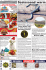### PDF - Entertainment Industries Council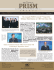### LED Full Color Digital Sign - Lichtkranten en Led Displays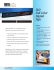### Lotus Speculos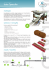### Vocabulary Cards 7th Grade N thru Z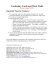### News/Talk/Sports Aircheck- June 2007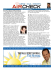### International Offshore Powerboat Race 2012 (Sarnia)